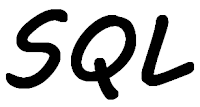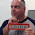## Wednesday, September 20, 2023

### New functions added to assist with Boolean data typeThe Boolean data type was introduced as part of IBM i 7.5, and does not work for lower releases.

With the last couple of Technology Releases, TRs, a number of SQL functions have been added to make using the Boolean data type easier. These are:

• BOOLEAN:  Returns Boolean value for parameter
• ISTRUE:  Returns "true" if the parameter is Boolean true, and if not returns "false" or null
• ISFALSE:  Returns "true" if the parameter is Boolean false, and "false" or null if it is not
• ISNOTTRUE:  Returns "true" if the parameter is not Boolean true, and returns "false" or null if not
• ISNOTFALSE:  Returns "true" if the parameter is not Boolean false, and if not then "false" or null

As I mentioned above I can use the BOOLEAN function to translate the value in the parameter to "true" or "false". For example I can use the function in a VALUES statement:

 ```01 VALUES (BOOLEAN(1),BOOLEAN(0),BOOLEAN('1'),BOOLEAN('0'), 02 BOOLEAN('true'),BOOLEAN(FALSE)) ```

Which returns:

 ```00001 00002 00003 00004 00005 00006 ----- ----- ----- ----- ----- ----- true false true false true false ```

The inability of being able to give columns headings with the Values statement can make the results difficult to understand as I have to refer to the SQL statement to determine what each column is.

The first, third, and fifth columns are all true as the parameters are logically "true".

Second, fourth, and sixth columns are "false".

What happens if a parameter is used that cannot be translated into Boolean value? I tried with:

 ```01 VALUES BOOLEAN('X') ```

"X" is not valid and cannot be translated, therefore, Run SQL Scripts errors with:

 ```SQL State: 22018 Vendor Code: -420 Message: [SQL0420] Value for cast argument not valid. ```

For the rest of my examples I need to have a SQL DDL that contains a Boolean type column:

 ```01 CREATE TABLE MYLIB.TESTTABLE 02 (COLUMN_1 BOOLEAN, 03 COLUMN_2 VARCHAR(10)) ; 04 INSERT INTO TESTTABLE 05 VALUES('TRUE','FIRST'), 06 ('FALSE','SECOND'), 07 (DEFAULT,'THIRD') ; ```

Lines 1 – 3: The definition of my table, called TESTTABLE. The definition for the Boolean type column is on line 2.

Lines 4 – 7: After the Table was created I need to insert data into it. This statement inserts three rows into the file. The third row inserts the default value into the Boolean column.

Using the following Select statement I can check the rows I inserted.

 ```SELECT * FROM TESTTABLE COLUMN_1 COLUMN_2 -------- -------- true FIRST false SECOND THIRD ```

The third row contains null in the Boolean column as that is the default for that column.

I now have data I can now use the other Boolean functions with:

 ```01 SELECT COLUMN_1, 02 ISTRUE(COLUMN_1) AS "ISTRUE", 03 ISFALSE(COLUMN_1) AS "ISFALSE", 04 ISNOTTRUE(COLUMN_1) AS "ISNOTTRUE", 05 ISNOTFALSE(COLUMN_1) AS "ISNOTFALSE" 06 FROM TESTTABLE ```

As COLUMN_1 is the Boolean type column all tests are performed upon it.

The results for the above is:

 ```COLUMN_1 ISTRUE ISFALSE ISNOTTRUE ISNOTFALSE -------- ------ ------- --------- ---------- true true false false true false false true true false false false true true ```

For the first row, which is Boolean "true", the results from the ISTRUE and ISNOTFALSE functions returned "true".

The second row, which is Boolean "false", the ISFALSE and ISNOTTRUE functions returned "true".

The third row is where the Boolean column is null. ISTRUE and ISFLASE functions return "false" as null is neither "true" nor "false". The ISNOTTRUE and ISNOTFALSE both return "true" as null is definitely not true or false.

I can get a count of each type of Boolean value in the Table with the following:

 ```01 SELECT COUNT(*) AS "Count" 02 FROM TESTTABLE 03 --WHERE ISTRUE(COLUMN_1) 04 --WHERE ISFALSE(COLUMN_1) 05 --WHERE ISNOTTRUE(COLUMN_1) 06 WHERE ISNOTFALSE(COLUMN_1) ```

Line 1: I need the COUNT(*) to count the number of rows.

Lines 3 – 6: I decided not to bore you with the result for each function. I have commented out the Where clause on lines 3 – 5, but have shown them so you know what the statement would look like if I had used that function. The results are expected:

• ISTRUE = 1 as there is only one Boolean "true" value
• ISFALSE = 1 as there is only one Boolean "false" value
• ISNOTTRUE = 2 as there is one Boolean "false" and one null
• ISNOTFALSE = 2 as there is one Boolean "true" and one null

I can do the same in a RPG program:

 ```01 **free 02 dcl-s Count uns(5) ; 03 Count = 0 ; 04 exec sql SELECT COUNT(*) INTO :Count 05 FROM TESTTABLE 06 WHERE ISTRUE(COLUMN_1) ; 07 dsply ('Count of ISTRUE = ' + %char(Count)) ; ```

Line 1: In 2023 all of our code should be totally free RPG.

Line 2: I have defined an unsigned integer variable to contain the result of the count. Well you can't have a count of less than zero or a fraction.

Line 3: In this program I did not have to do this. In another program where I am using this variable multiple times if I do not initialize it and the following statement fails the variable is unchanged, containing the value from the last time it was used. I always initialize the variable to make sure that if the Select fails the variable contains zero.

Lines 4 – 6: The INTO on the fourth line means that the result of the count is place in the RPG variable Count. Here I want a count of all the rows where the Boolean column is "true". If no records are found the RPG variable is not updated, which is why I do what I did on line 3.

Line 7: I am using the Display operation code, DSPLY, to display the count value to me.

The count of "true" rows is:

 ```DSPLY Count of ISTRUE = 1 ```

In my next example I want to retrieve from the table all the rows where COLUMN_1 is not false. This I do with a SQL Cursor:

 ```01 **free 02 dcl-ds Data extname('TESTTABLE') qualified dim(*auto : 10) ; 03 end-ds ; 04 dcl-ds DataNull qualified dim(*auto : 10) ; 05 Ind int(5) dim(2) ; 06 end-ds ; 07 dcl-s Elements uns(5) inz(%elem(Data : *max)) ; 08 exec sql DECLARE C0 CURSOR FOR 09 SELECT * FROM TESTTABLE 10 WHERE ISNOTFALSE(COLUMN_1) 11 FOR READ ONLY ; 12 exec sql OPEN C0 ; 13 exec sql FETCH C0 FOR :Elements ROWS INTO :Data :DataNull ; 14 exec sql CLOSE C0 ; ```

Lines 2 and 3: This is the data structure array definition the data from TESTTABLE will be placed into. I don't need to define any data structure sub-fields as the definition is taken from the file or table in the EXTNAME keyword. This is also an auto extending array that can have a maximum of ten elements.

Lines 4 – 6: I cannot convert any nulls in COLUMN_1 by using the IFNULL function as that would give me misleading results. What I need to do is to capture which columns are null. I do this by using SQL null indicators. These are not indicators in the RPG sense. SQL null indicators are 5 long integers. I need to define one of these indicators for each column in the table. As there are two columns in TESTABLE I need an array of two elements, line 5. This array is contained within a data structure array, line 4, which needs to contain the same number of elements as the first data structure array.

Line 7: I am defining variable to contain the maximum number of elements in the first array.

Lines 8 – 11: This is the definition of the cursor to retrieve all of the rows from TESTTABLE where COLUMN_1 is not "false".

Line 12: I open the cursor.

Line 13: I retrieve rows from the cursor up to a maximum of the number of in the variable Elements. In this scenario there are only two results. I am moving my results into the two data structure arrays. Notice there is no comma between the two. The Fetch will place the data from the rows into Data and the nulls in the form of null indicators into DataNull.

Line 14: I close the cursor.

If I place a debug break point after I have closed the cursor and I look at the contents of Data, I see I have two array elements of data:

 ```> EVAL data DATA.COLUMN_1(1) = '1' DATA.COLUMN_2(1) = 'FIRST ' DATA.COLUMN_1(2) = ' ' DATA.COLUMN_2(2) = 'THIRD ' DATA.COLUMN_1(3) = ' ' DATA.COLUMN_2(3) = ' ' ```

Notice that DATA.COLUMN_1(2), COLUMN_1 from the second result row, contains blank, when it could be expected to some how show null. This is where the second data structure array, for the null indicators, comes into play:

 ```> EVAL datanull DATANULL.IND(1,1) = 0 DATANULL.IND(1,2) = 0 DATANULL.IND(2,1) = -1 DATANULL.IND(2,2) = 0 DATANULL.IND(3,1) = 0 DATANULL.IND(3,2) = 0 ```

DATANULL.IND(2,1) contains the null indicator for the second row that was retrieved first column, which is COLUMN_1. As this contains the value of -1 the column for that row is null.

These functions make checking for values in the Boolean columns easier than it would be without.

1.It is really stupid we need functions to work with boolean columns.
The idea was to use them as (examples):
"if boolean_column then...",
"if not boolean_column and other_column>5 then..."
As in any other language.

2.1. These Boolean functions were part of the initial IBM i 7.5 release.
2. SQL logic dealing with Boolean data can be written several ways. Use what you are most comfortable with. For example:
WHERE boolcol
WHERE boolcol is true
WHERE boolcol = true
WHERE istrue(boolcol)
3. UNKNOWN is a synonym for NULL, aligning with Boolean logic concepts.
WHERE boolcol is null
WHERE boolcol is unknown

1.To prevent "comment spam" all comments are moderated.
```